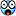Welcome to Doom9's Forum, THE in-place to be for everyone interested in DVD conversion. Before you start posting please read the forum rules. By posting to this forum you agree to abide by the rules.Doom9's Forumscramble/descramble: help needed
 Register FAQ Calendar Search Today's Posts Mark Forums Read2nd March 2019, 23:17 #1  |  Link spoRv Registered User   Join Date: Nov 2016 Posts: 114 scramble/descramble: help needed I'd like to develop a function to scramble and descramble video; the idea is to "shuffle" video enough to let it not be (easily) recognizable. I ended up with a simple function that divides the image in small blocks, shuffle them, invert/flip etc. At the moment, it is VERY basic, but serves as a starting point to show how the function works: Code: ```function scramble(clip clip) { w=clip.width/4 h=clip.height/4 clip a01=crop(0 ,0 ,w,h).fliphorizontal a02=crop(w ,0 ,w,h).invert("br") a03=crop(w*2,0 ,w,h).invert a04=crop(w*3,0 ,0,h).turn180 a05=crop(0 ,h ,w,h).invert a06=crop(w ,h ,w,h).flipvertical a07=crop(w*2,h ,w,h).invert a08=crop(w*3,h ,0,h).invert("rg") a09=crop(0 ,h*2,w,h).swapuv a10=crop(w ,h*2,w,h).turn180 a11=crop(w*2,h*2,w,h).swapuv a12=crop(w*3,h*2,0,h).turn180 a13=crop(0 ,h*3,w,0).swapuv a14=crop(w ,h*3,w,0).invert("gb") a15=crop(w*2,h*3,w,0).flipvertical a16=crop(w*3,h*3,0,0).fliphorizontal stackvertical(\ stackhorizontal(a12,a05,a08,a15),\ stackhorizontal(a06,a10,a01,a09),\ stackhorizontal(a11,a16,a14,a04),\ stackhorizontal(a03,a13,a07,a02)) }``` Code: ```function descramble(clip clip) { w=clip.width/4 h=clip.height/4 clip a01=crop(0 ,0 ,w,h) a02=crop(w ,0 ,w,h) a03=crop(w*2,0 ,w,h) a04=crop(w*3,0 ,0,h) a05=crop(0 ,h ,w,h) a06=crop(w ,h ,w,h) a07=crop(w*2,h ,w,h) a08=crop(w*3,h ,0,h) a09=crop(0 ,h*2,w,h) a10=crop(w ,h*2,w,h) a11=crop(w*2,h*2,w,h) a12=crop(w*3,h*2,0,h) a13=crop(0 ,h*3,w,0) a14=crop(w ,h*3,w,0) a15=crop(w*2,h*3,w,0) a16=crop(w*3,h*3,0,0) stackvertical(\ stackhorizontal(a07,a16,a13,a12),\ stackhorizontal(a02,a05,a15,a03),\ stackhorizontal(a08,a06,a09,a01),\ stackhorizontal(a14,a11,a04,a10)) a01=crop(0 ,0 ,w,h).fliphorizontal a02=crop(w ,0 ,w,h).invert("br") a03=crop(w*2,0 ,w,h).invert a04=crop(w*3,0 ,0,h).turn180 a05=crop(0 ,h ,w,h).invert a06=crop(w ,h ,w,h).flipvertical a07=crop(w*2,h ,w,h).invert a08=crop(w*3,h ,0,h).invert("rg") a09=crop(0 ,h*2,w,h).swapuv a10=crop(w ,h*2,w,h).turn180 a11=crop(w*2,h*2,w,h).swapuv a12=crop(w*3,h*2,0,h).turn180 a13=crop(0 ,h*3,w,0).swapuv a14=crop(w ,h*3,w,0).invert("gb") a15=crop(w*2,h*3,w,0).flipvertical a16=crop(w*3,h*3,0,0).fliphorizontal stackvertical(\ stackhorizontal(a01,a02,a03,a04),\ stackhorizontal(a05,a06,a07,a08),\ stackhorizontal(a09,a10,a11,a12),\ stackhorizontal(a13,a14,a15,a16)) }``` What I'd like to reach is the following: - input a N number, where the image will be divided in N x N parts - I guess N <= 16 is enough - input a string with N triplets, where first character is the X, second is the Y, and third is the type of "manipulation" of that given sector (like, for example, V = flipvertical, I = invert etc. and of course a combination of more than a single one) - possibly get a random string with a further function I know that it's possible to use scriptclip to reiterate the stacking, and midstr to get the string triplets, but I do not know how to put them in use!Note: it works with uncompressed/lossless video, I'm pretty sure it will NOT work with lossy video. If someone has a better/different way to reach the target - shuffle the image as much as possible, getting the file size not so much bigger - it will be great! As usual, thanks in advance to anybody can help!Tags descramble, scrambleThread Tools Search this ThreadShow Printable VersionEmail this Page Search this Thread: Advanced Search Display ModesSwitch to Linear ModeSwitch to Hybrid ModeThreaded ModePosting Rules You may not post new threads You may not post replies You may not post attachments You may not edit your posts BB code is On Smilies are On [IMG] code is On HTML code is Off Forum Rules
 Forum Jump User Control Panel Private Messages Subscriptions Who's Online Search Forums Forums Home Announcements and Chat     General Discussion     News     Forum / Site Suggestions & Help General     Decrypting     Newbies     DVD2AVI / DGIndex     Audio encoding     Subtitles     Linux, Mac OS X, & Co Capturing and Editing Video     Avisynth Usage     Avisynth Development     VapourSynth     Capturing Video     DV     HDTV / DVB / TiVo     NLE - Non Linear Editing     VirtualDub, VDubMod & AviDemux     New and alternative a/v containers Video Encoding     (Auto) Gordian Knot     MPEG-4 ASP     MPEG-4 Encoder GUIs     MPEG-4 AVC / H.264     High Efficiency Video Coding (HEVC)     New and alternative video codecs     MPEG-2 Encoding (HD) DVD, Blu-ray & (S)VCD     One click suites for DVD backup and DVD creation     DVD & BD Rebuilder     (HD) DVD & Blu-ray authoring     Advanced authoring     IFO/VOB Editors     DVD burning Hardware & Software     Software players     Hardware players     PC Hard & Software Programming and Hacking     Development     Translations

All times are GMT +1. The time now is 10:04.

 Doom9.org - Archive - Top